2021 NFL MVP Odds
+350
3.5 to 1Tom Brady
19.0% implied probability

+500
5 to 1Josh Allen
14.3% implied probability

+600
6 to 1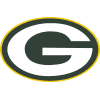Aaron Rodgers
12.2% implied probability

+1000
10 to 1Dak Prescott
7.8% implied probability

+1200
12 to 1Patrick Mahomes
6.6% implied probability

+1200
12 to 1Kyler Murray
6.6% implied probability

+1800
18 to 1Matthew Stafford
4.5% implied probability

+1800
18 to 1Lamar Jackson
4.5% implied probability

+1800
18 to 1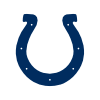Jonathan Taylor
4.5% implied probability

+1800
18 to 1Justin Herbert
4.5% implied probability

+3500
35 to 1Cooper Kupp
2.4% implied probability

+4000
40 to 1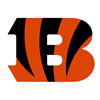Joe Burrow
2.1% implied probability

+4000
40 to 1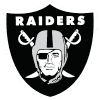Derek Carr
2.1% implied probability

+5000
50 to 1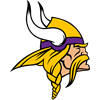Kirk Cousins
1.7% implied probability

+10000
100 to 1Austin Ekeler
0.8% implied probability

+10000
100 to 1Jalen Hurts
0.8% implied probability

+10000
100 to 1Teddy Bridgewater
0.8% implied probability

+10000
100 to 1Mac Jones
0.8% implied probability

+10000
100 to 1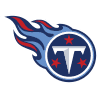Ryan Tannehill
0.8% implied probability

+10000
100 to 1James Conner
0.8% implied probability

+20000
200 to 1Joe Mixon
0.4% implied probability

+20000
200 to 1Carson Wentz
0.4% implied probability

+20000
200 to 1Deebo Samuel
0.4% implied probability

+25000
250 to 1Tyreek Hill
0.3% implied probability

+25000
250 to 1Justin Jefferson
0.3% implied probability

+50000
500 to 1Davante Adams
0.2% implied probability